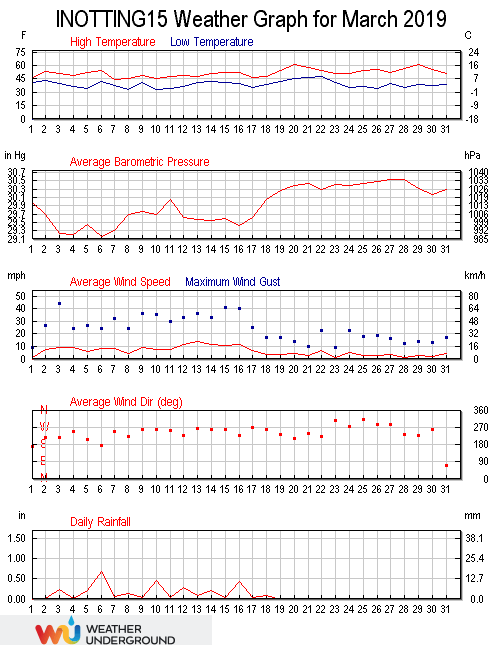March 2019 was warmer than average (7.4°C versus an average of 5.8°C), wetter than average (77.60 mm versus an average total of 40.56) and windier than average (a wind run of 4495 miles versus an average of 3112). March was a month of two distinct halves, the first 18 days being cold, damp and windy, with the final 13 days being milder and dry.

The highest temperature in the month was 29th at 15:43 when 16.2°C was recorded. The lowest temperature was 0.6°C recorded at 07:00 on the 10th. This is the highest March minimum ever recorded here. The average temperature in the month was 7.4°C, 1.4°C above the mean. The average daily high temperature was 10.9°C, mean 9.9°C, and the average daily low temperature was 3.9°C, mean 2.6°C. The warmest day was the 20th with an average temperature of 11.0°C, while the coldest day was the 10th with an average temperature of 3.1°C.

The monthly average wind chill was 5.8°C. The high dew point was 11.8°C at 13:12 on the 20th, with the low dew point being -1.1°C at 16:15 on the 10th. The monthly average dew point was 4.4°C. There were 120.3 hours of sunshine in the month, the sunniest day being the 29th when 8.88 hours was recorded.

Rainfall in the month totalled 77.6 millimetres, well above the average of 43.7 milliemtres. The year to date total of 128.8 millimetres is 106% of the expected March end total. The wettest day was 6th with 17.6 millimetres, making it the wettest day since September 20th 2018 when 19.4 millimetres was recorded.. All the month’s rainfall falling on the first 18 days. The highest rain rate occured at 18:33 on the 6th when a rate of 50 millimetres per hour was recorded. Measurable snow was recorded on the 10th of the month only and totalled 15.0 millimetres.

The highest barometric pressure of 1036.1 millibars was at 07:14 on the 28th, with the lowest being 979.4 millibars at 22:05 on the 3rd. The average pressure in the month was 1012.9 millibars. The highest humidity was 98% at 07:38 on the 21st, and the monthly average humidity was 82%. The lowest humidity was 49% at 16:00 on the 25th.

Winds were stronger than average in the month, with the highest wind gust speed being 44 mph recorded at 21:54 on the 3rd, well the month average of 35.7 mph. This was the highest gust since 50 mph was recorded on February 23rd 2017. The maximum wind speed was 24 mph at 11:18 on the 15th, 2.2 mph above the mean. The wind run was 4495.3 miles, well above the March average of 3532.9 miles. The average gust speed in the month was 8.7 mph, 1.3 mph above the mean. The average wind speed was 6.2 mph, 1.5 mph above the mean. The predominant direction was south westerly (220°).

The maximum high solar radiation recorded at 12:00 on the 24th was 907 w/m², the highest since 914 w/m² was recorded on September 21st 2018. The average solar radiation for the month was 106 w/m². The highest U.V. Index was 2.8 recorded at 11:45 on the 19th, the highest since 2.9 was recorded on September 27th 2018.This site uses Akismet to reduce spam. Learn how your comment data is processed.